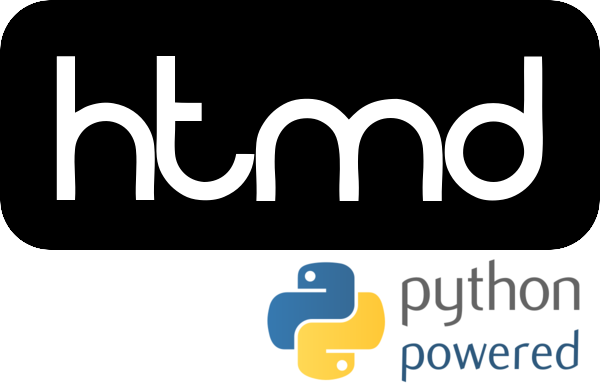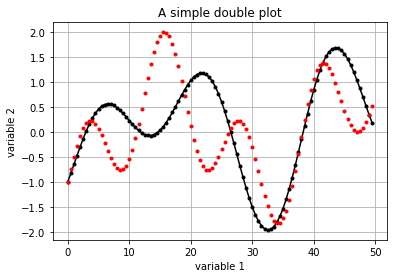# Python primer for HTMD### Why Python?#

• Modern, complete and open-source programming language

• Wide spread throughout the scientific community

• Easy to understand, rapid delivery and maintenance

• C++ still wins on low latency and control of memory

• However, Python is versatile and has packages that can be as fast as C (e.g. NumPy)

## HTMD relation to Python and Miniconda#

• HTMD is written in Python, using the Python 3 synthax

• HTMD is, and works as, a Python module.

• HTMD is distributed through a package/module distribution platform called Miniconda (light-weight Anaconda)

1. Already distributes Python (`python`) and interactive Python (`ipython`) interpreters

2. Everything is self-contained inside Miniconda and one does not have to rely on the operative system

## Programming basics with Python#

• Python can do pretty much what any other programming language can. Arithmetics, variable definition, printing, etc.

```a = 3 + 2
print('a is', a)
```
```a is 5
```

## Python Interpreters and writing Python code#

• `python` is the general interpreter

• One can write a `script.py` file in Python and run `python script.py`

• There are powerful ways of writing and prototyping code in Python:

• Interactive interpreters (`ipython`, `jupyter`)

• IDE’s (Spyder, Pycharm)

## Jupyter Notebooks#

• This presentation is actually a Jupyter notebook (you can play with it, run the code yourself)

• A notebook like this one is a grapical interactive environment.

• It is made of cells:

• Text cells, with formatted markdown text (like the bulleted text we have here)

• Code cells, to run code interactively

### Extra: IPython built-in magic commands#

• In IPython, some magic commands are available/built-in.

• Useful to navigate the filesystem (% indicates a magic command)

```%ls
%mkdir test
```
• Or for module reload for rewritting code for testing without need to reload the module all the time

```%load_ext autoreload
```

## Python modules usage#

• A Python module is a file containing Python definitions and statements.

• In Python, modules have to be imported in order to be used.

• Here we show two ways of importing modules and one way of importing a functionality:

```import os
import numpy as np
from glob import glob
```

In the first two, using the module funcionalities require the namespace: the original one `os`, or the defined one `np` (instead of `numpy`). In the last one, `glob` can be used instead of `glob.glob`

## `os` module and `glob` function: interacting with the filesystem#

• The `os` module serves to interact with the operative system

• Normal filesystem commands or magic commands work from the interactive session, but, in a program, the `os` functions should be used instead

```os.chdir('.') # change directory
os.listdir('.') # list contents of directory
glob('python-*') # returns pathnames matching a regex pattern
```
```['python-primer.ipynb', 'python-primer.slides.html']
```

## General Programming Overview#

### Strings#

Strings are identified by single or double apices:

```b='newtest'
c="newtest"
```

### Relational operators#

Relational operators are `==`,`<`,`>`,`<=`,`>=`, and `!=`, and they can be connected with `and`,`or`,`not`

```b == c
```
```True
```

### Lists of objects (integers, strings…)#

Lists of different objects are created using square brackets

```d = [1, 2, 3, 'ba', 5, 6, 7, 8, 9]
print(d)
```
```ba
```

Note: in Python, indexes are zero-based.

### Conditionals (if-clause)#

```if 1 > 0:
d = 100
print(d)
```
```100
```

As you probably know, in Python, indentation is important. Use indentation to define a scope of code after the `:`. `print(d)` is no longer inside the scope of the if-clause because it is not indented.

### Loops (for-loop)#

```for i in [1,2,3,4,5]:
print(i)
```
```1
2
3
4
5
```

Another way of doing the same, using Numpy:

```for i in np.arange(1,6):
print(i)
```
```1
2
3
4
5
```

## Numpy: the best way to handle arrays#

Arrays are best created and handled using `numpy` arrays (no need to import the module, we already did before)

```m = np.array([[ 11., 12, 13, 14 ],
[ 21, 22, 23, 24 ],
[ 31, 32, 33, 34 ],
[ 41, 42, 43, 44 ]
]
)
print(m)
```
```[[ 11.  12.  13.  14.]
[ 21.  22.  23.  24.]
[ 31.  32.  33.  34.]
[ 41.  42.  43.  44.]]
```

where `m` is 4-by-4 matrix of double numbers (and not integers), due to the fact than `11.` is a real number (notice the dot).

### Array multiplication#

Arrays can be multiplied easily element by element

```n = 3.0 * m
print(n)
```
```[[  33.   36.   39.   42.]
[  63.   66.   69.   72.]
[  93.   96.   99.  102.]
[ 123.  126.  129.  132.]]
```

A scalar multiplication is applied to each element of the array.

### Sequences#

An array sequence can be created with `arange` (like seen before). Array indexing starts at 0.

```o = np.arange(0,4)
print(o)
```
```[0 1 2 3]
```

### Slicing#

```o[1:] #from 1 until the end, (starts at zero)
```
```array([1, 2, 3])
```

Note: See how we got the outcome without printing? It’s because we’re in interactive Python

```m[:,0] = 99 # first column
m[-2:,]     # backwards indexing is possible, getting the last 2 rows
```
```array([[ 99.,  32.,  33.,  34.],
[ 99.,  42.,  43.,  44.]])
```

### More matrix operations#

```np.multiply(m,o)
```
```array([[   0.,   12.,   26.,   42.],
[   0.,   22.,   46.,   72.],
[   0.,   32.,   66.,  102.],
[   0.,   42.,   86.,  132.]])
```
```m.transpose()
np.inner(m,o)
```
```array([  80.,  140.,  200.,  260.])
```

### Other numpy operations#

```np.concatenate((o,o))
```
```array([0, 1, 2, 3, 0, 1, 2, 3])
```
```m.sum(axis=0)
```
```array([ 396.,  108.,  112.,  116.])
```
```print(o.shape)
print(o.size)
print(o.ndim)
```
```(4,)
4
1
```

## Functions#

Subroutines are defined using `def`:

```def test(a, b=1, c=3):
return a*b*c
```
```test(1, c=5)
```
```5
```

## Variables and Objects#

• Python represents all its data as objects.

• Variables are just names.

• Some objects are mutable, some immutable.

• Immutables are: int, float, complex, str, tuples, bytes, frozensets

• Mutables are: list, byte array, sets, dict, classes

## Identity of an object#

With `id` it is possible to check the unique identity of an object

```n = 1 # immutable integer
id(n)
```
```140351964294272
```
```n += 1
id(n) # new object
```
```140351964294304
```
```m =  # mutable list
id(m)
```
```140351546176392
```
```m.append(2)
id(m) # same object
```
```140351546176392
```

## Argument passing#

• Passing an argument to a function is like creating a new name to the object

• If it is mutable, then any change inside the function will affect the object outside.

• If it is immutable and the function changes it, then python creates another object inside the function scope, so nothing changes outside of the function.

## Plots using `matplotlib` module#

```from matplotlib.pylab import plot,title,xlabel,ylabel,grid,show,savefig,gcf
#Optional: magic line to make plots inline
%matplotlib inline

x = np.arange(0,50,.5)
y = np.sin(x/3) - np.cos(x/5)
plot(x,y, '.-k')
plot(x,np.sin(x/2) - np.cos(x/5),'.r')
title('A simple double plot')
xlabel('variable 1'), ylabel('variable 2'), grid(True)
fig = gcf() # Get current figure
show()
```The plot is shown inline, but can also be saved:

```fig.savefig('/tmp/foo.eps')
```

## Getting Help#

The `help` function provides help for any function:

```help(print)
```
```Help on built-in function print in module builtins:

print(...)
print(value, ..., sep=' ', end='n', file=sys.stdout, flush=False)

Prints the values to a stream, or to sys.stdout by default.
Optional keyword arguments:
file:  a file-like object (stream); defaults to the current sys.stdout.
sep:   string inserted between values, default a space.
end:   string appended after the last value, default a newline.
flush: whether to forcibly flush the stream.```

## Debugging code in notebooks#

Really nice to debug an error and see what’s wrong by inspecting variables using `%debug` (Press PgDown for example)

```def broken_function(b):
print(b,xxx())
c = 4
broken_function(c)
```
```---------------------------------------------------------------------------

NameError                                 Traceback (most recent call last)

<ipython-input-29-441f8be6e164> in <module>()
2     print(b,xxx())
3 c = 4
----> 4 broken_function(c)

<ipython-input-29-441f8be6e164> in broken_function(b)
1 def broken_function(b):
----> 2     print(b,xxx())
3 c = 4
4 broken_function(c)

NameError: name 'xxx' is not defined
```
```%debug
```
```> [0;32m<ipython-input-29-441f8be6e164>[0m(2)[0;36mbroken_function[0;34m()[0m
[0;32m      1 [0;31m[0;32mdef[0m [0mbroken_function[0m[0;34m([0m[0mb[0m[0;34m)[0m[0;34m:[0m[0;34m[0m[0m
[0m[0;32m----> 2 [0;31m    [0mprint[0m[0;34m([0m[0mb[0m[0;34m,[0m[0mxxx[0m[0;34m([0m[0;34m)[0m[0;34m)[0m[0;34m[0m[0m
[0m[0;32m      3 [0;31m[0mc[0m [0;34m=[0m [0;36m4[0m[0;34m[0m[0m
[0m[0;32m      4 [0;31m[0mbroken_function[0m[0;34m([0m[0mc[0m[0;34m)[0m[0;34m[0m[0m
[0m
ipdb> quit
```

## Python Exercises#

1. Sum the first 50 numbers with a for loop

2. Do the same thing using `numpy` arrays

3. Write a function that set a value for its argument (that is an integer)

4. The same but for a `numpy` arrray